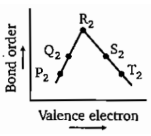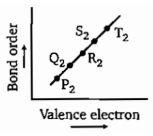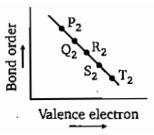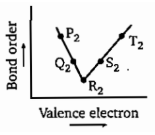The radius of which ion is closest to that of $L{i}^{+}$ ion?

1. $N{a}^{+}$                                        2. $B{e}^{2+}$

3. $M{g}^{2+}$                                       4. $A{l}^{3+}$

Concept Questions :-

Atomic Size
High Yielding Test Series + Question Bank - NEET 2020

Difficulty Level:

The correct order of $I{E}_{2}$ is:

(a) Na>F>O>N                      (b) O>F>Ne>N
(c) Ne>O>F>N                      (d) O>Ne>F>N

Concept Questions :-

Ionization Energy (IE)
High Yielding Test Series + Question Bank - NEET 2020

Difficulty Level:

Which of the following transformation least energy is required?

(a)                   (b) ${{P}^{-}}_{\left(g\right)}\to {P}_{\left(g\right)}+{e}^{-}$

(c) ${{S}^{-}}_{\left(g\right)}\to {S}_{\left(g\right)}+{e}^{-}$                    (d) $C{{l}^{-}}_{\left(g\right)}\to C{l}_{\left(g\right)}+{e}^{-}$

Concept Questions :-

Electron Affinity (EA)
High Yielding Test Series + Question Bank - NEET 2020

Difficulty Level:

Which of the following represents correct order of electron affinity?

(a) Cl>F>S>O                            (b) F>O>S>Cl

(c) F>Cl>S>O                            (d) Cl>S>O>F

Concept Questions :-

Electron Affinity (EA)
High Yielding Test Series + Question Bank - NEET 2020

Difficulty Level:

Correct expression of "Allred and Rochow's" scale is:

(a) Electronegativity =$0.744\frac{{Z}_{eff.}}{{r}^{2}}+0.359$

(b) Electronegativity =$0.359\frac{{r}^{2}}{{Z}_{eff.}}+0.744$

(c) Electronegativity =$0.359\frac{{Z}_{eff.}}{r}+0.744$

(d) Electronegativity =$0.359\frac{{Z}_{eff.}}{{r}^{2}}+0.744$

Concept Questions :-

Electronegativity
High Yielding Test Series + Question Bank - NEET 2020

Difficulty Level:

The process requiring absorption of energy is:

(a) $N\to {N}^{-}$                       (b) $F\to {F}^{-}$

(c) $Cl\to C{l}^{-}$                      (d) $H\to {H}^{-}$

Concept Questions :-

Electron Affinity (EA)
High Yielding Test Series + Question Bank - NEET 2020

Difficulty Level:

Which of the following compounds has a positive enthalpy of solution?

(a) LiF                                         (b) LiCl

(c) LiBr                                        (d) Lil

Concept Questions :-

Lattice/Hydration Energy
High Yielding Test Series + Question Bank - NEET 2020

Difficulty Level:

Which of the following compound is most acidic?

(a) $C{l}_{2}{O}_{7}$                              (b) ${P}_{4}{O}_{10}$

(c) $S{O}_{3}$                                 (d) ${B}_{2}{O}_{3}$

Concept Questions :-

Nature of Compound
High Yielding Test Series + Question Bank - NEET 2020

Difficulty Level:

If P and T are second period p-block elements then which of the following graph show correct relation between valence electrons in ${P}_{2}$ to ${T}_{2}$ (corresponding molecules) and their bond order is :(a)                                                                                    (b)(c)                                                                                     (d)

Concept Questions :-

Modern Periodic Table
High Yielding Test Series + Question Bank - NEET 2020

Difficulty Level:

A compound contains three elements A, B and C, if the oxidation number of A =+2, B =+5 and C =-2, the possible formula of the compound is :

(a) ${A}_{3}{\left({B}_{4}C\right)}_{2}$                                  (b) ${A}_{3}{\left(B{C}_{4}\right)}_{2}$

(c) ${A}_{2}{\left(B{C}_{3}\right)}_{2}$                                  (d) $AB{C}_{2}$

Concept Questions :-

Nature of Compound